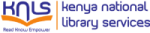Normal view

# Continuous signals and systems with MATLAB / Taan S. ElAli.

Material type:TextSeries: Publisher: Boca Raton, FL : CRC Press, Edition: Third editionDescription: 1 online resource (xv, 345 pages) : illustrationsContent type:
• text
Media type:
• computer
Carrier type:
• online resource
ISBN:
• 9781000180015
• 1000180018
• 9781000179972
• 1000179974
• 9781003088585
• 1003088589
DDC classification:
• 621.382/2 23
LOC classification:
• TK5102.9 .E34 2021
Online resources: Summary: Continuous Signals and Systems with MATLAB offers broad, detailed, and focused comprehensive coverage of continuous linear systems, based on basic mathematical principles. It presents many solved problems from various engineering disciplines using analytical tools as well as MATLAB. This book is intended primarily for undergraduate junior and senior electrical, mechanical, aeronautical, and aerospace engineering students. Practicing engineers will also find this book useful. This book is ideal for use in a one-semester course in continuous linear systems where the instructor can easily cover all of the chapters. Each chapter presents numerous examples that illustrate each concept. Most of the worked-out examples are first solved analytically, and then solved using MATLAB in a clear and understandable fashion. This book concentrates on explaining the subject matter with easy-to-follow mathematical development and numerous solved examples. The book covers traditional topics and includes an extensive coverage of state-space representation and analysis. The reader does not need to be fluent in MATLAB because the examples are presented in a self-explanatory way.
Tags from this library: No tags from this library for this title.
Star ratingsAverage rating: 0.0 (0 votes)
No physical items for this record

Previous edition: 2008.

<P>Chapter 1 Signal Representation<BR>1.1 Examples of Continuous Signals<BR>1.2 The Continuous Signal<BR>1.3 Periodic and Nonperiodic Signals<BR>1.4 General Form of Sinusoidal Signals<BR>1.5 Energy and Power Signals<BR>1.6 The Shifting Operation<BR>1.7 The Reflection Operation<BR>1.8 Even and Odd Functions <BR>1.9 Time Scaling<BR>1.10 The Unit Step Signal <BR>1.11 The Signum Signal<BR>1.12 The Ramp Signal<BR>1.13 The Sampling Signal <BR>1.14 The Impulse Signal<BR>1.15 Some Insights: Signals in the Real World<BR>1.16 End-of-Chapter Examples <BR>1.17 End-of-Chapter Problems<BR>References</P><P><BR>Chapter 2 Continuous Systems<BR>2.1 Definition of a System<BR>2.2 Input and Output<BR>2.3 Linear Continuous System<BR>2.4 Time-Invariant System<BR>2.5 Systems without Memory<BR>2.6 Causal Systems<BR>2.7 The Inverse of a System<BR>2.8 Stable Systems<BR>2.9 Convolution<BR>2.10 Simple Block Diagrams<BR>2.11 Graphical Convolution<BR>2.12 Differential Equations and Physical Systems <BR>2.13 Homogeneous Differential Equations and Their Solutions<BR>2.14 Nonhomogeneous Differential Equations and Their Solutions<BR>2.15 The Stability of Linear Continuous Systems: The Characteristic Equation<BR>2.16 Block Diagram Representation of Linear Systems<BR>2.17 From Block Diagrams to Differential Equations <BR>2.18 From Differential Equations to Block Diagrams <BR>2.19 The Impulse Response<BR>2.20 Some Insights: Calculating y(t)<BR>2.21 End-of-Chapter Examples<BR>2.22 End-of-Chapter Problems<BR>References</P><P><BR>Chapter 3 Fourier Series<BR>3.1 Review of Complex Numbers<BR>3.2 Orthogonal Functions <BR>3.3 Periodic Signals<BR>3.4 Conditions for Writing a Signal as a Fourier Series Sum<BR>3.5 Basis Functions<BR>3.6 The Magnitude and the Phase Spectra<BR>3.7 Fourier Series and the Sin-Cos Notation<BR>3.8 Fourier Series Approximation and the Resulting Error<BR>3.9 The Theorem of Parseval<BR>3.10 Systems with Periodic Inputs<BR>3.11 A Formula for Finding y(t) When x(t) Is Periodic: The Steady-State Response<BR>3.12 Some Insight: Why the Fourier Series?<BR>3.13 End-of-Chapter Examples<BR>3.14 End-of-Chapter Problems<BR>References</P><P><BR>Chapter 4 The Fourier Transform and Linear Systems<BR>4.1 Definition<BR>4.2 Introduction<BR>4.3 The Fourier Transform Pairs<BR>4.4 Energy of Non-Periodic Signals<BR>4.5 The Energy Spectral Density of a Linear System<BR>4.6 Some Insights: Notes and a Useful Formula<BR>4.7 End-of-Chapter Examples<BR>4.8 End-of-Chapter Problems<BR>References<BR></P><P>Chapter 5 The Laplace Transform and Linear Systems<BR>5.1 Definition <BR>5.2 The Bilateral Laplace Transform<BR>5.3 The Unilateral Laplace Transform<BR>5.4 The Inverse Laplace Transform<BR>5.5 Block Diagrams Using the Laplace Transform<BR>5.6 Representation of Transfer Functions as Block Diagrams<BR>5.7 Procedure for Drawing the Block Diagram from the Transfer Function<BR>5.8 Solving LTI Systems Using the Laplace Transform<BR>5.9 Solving Differential Equations Using the Laplace Transform<BR>5.10 The Final Value Theorem<BR>5.11 The Initial Value Theorem<BR>5.12 Some Insights: Poles and Zeros <BR>5.13 End-of-Chapter Examples <BR>5.14 End-of-Chapter Problems<BR>References</P><P><BR>Chapter 6 State-Space and Linear Systems<BR>6.1 Introduction<BR>6.2 A Review of Matrix Algebra<BR>6.3 General Representation of Systems in State-Space<BR>6.4 General Solution of State-Space Equations Using the Laplace Transform<BR>6.5 General Solution of the State-Space Equations in Real Time<BR>6.6 Ways of Evaluating eAt <BR>6.7 Some Insights: Poles and Stability<BR>6.8 End-of-Chapter Examples<BR>6.9 End-of-Chapter Problems<BR>References</P>

Continuous Signals and Systems with MATLAB offers broad, detailed, and focused comprehensive coverage of continuous linear systems, based on basic mathematical principles. It presents many solved problems from various engineering disciplines using analytical tools as well as MATLAB. This book is intended primarily for undergraduate junior and senior electrical, mechanical, aeronautical, and aerospace engineering students. Practicing engineers will also find this book useful. This book is ideal for use in a one-semester course in continuous linear systems where the instructor can easily cover all of the chapters. Each chapter presents numerous examples that illustrate each concept. Most of the worked-out examples are first solved analytically, and then solved using MATLAB in a clear and understandable fashion. This book concentrates on explaining the subject matter with easy-to-follow mathematical development and numerous solved examples. The book covers traditional topics and includes an extensive coverage of state-space representation and analysis. The reader does not need to be fluent in MATLAB because the examples are presented in a self-explanatory way.